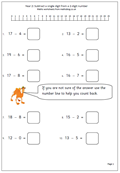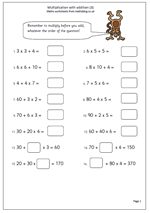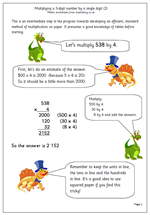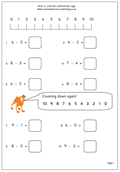## Calculating in Year 2In Year 2 children will be calculating using the addition, subtraction, multiplication and division symbols. Their understanding of addition will develop as they begin to add a 1-digit number to a 2-digit number as well as beginning to answer written addition questions. (This is not the same as using written methods.) Developing efficient mental methods of addition and subtraction are still the most important aspects here, as written methods, apart from jottings, should not yet be used.

Subtraction is much easier once it is realised that it is the inverse (or opposite) of addition. Many subtraction questions can be answered by adding on, but children are expected to know subtraction facts to at least 10 (eg know that 9 – 4 = 5). Knowing these facts is just as important as knowing ‘tables’. They will also be working with 2-digit numbers, especially multiples of 10.

There is a good selection of addition and subtraction worksheets on the site in our Year 2 calculating section as well as a growing number of multiplication and division pages. Multiplication is seen as repeated addition and the 2x, 5x and 10x tables will be learned during the year.

As well as in the Calculating section further worksheets can be found in Knowing Number Facts and our Four Rules section.

Go to Year 2 calculating worksheets

## Year 4 CalculatingPart of the site which is developing well and is well worth a look is the Year 4 Calculating category. There is much to be learnt in year 4, including adding three small numbers mentally and being able to add two 2-digit numbers ‘in your head’. There are a lot of strategies which need to be understood and become second nature to make this happen including partitioning numbers. It is also important to understand the relationship between addition and subtraction, which is very useful to use when checking answers.

Year 4 is a time for consolidating knowledge of tables but other ideas are also introduced including number sentences with more than one operation (later leading to BODMAS).

Division becomes much easier when times tables are known and a lot of the work on division in Year 4 involves remainders and writing remainders as fractions.

Why not have a look at our year 4 calculating worksheets? There are still more in the Four Rules section of the site.

Year 4 Calculating

## Year 5 CalculatingThere is a great deal expected of children in year 5 when it comes to calculating. Mental methods of calculating with whole numbers are extended to include addition of three small numbers, knowing tables and multiplying by 25. Efficient written methods of addition and subtraction of both whole numbers and decimals is expected as well as refining written methods of multiplication and division.

I have a growing range of worksheets covering these topics which can be found in the Year 5 Calculating category. Further worksheets on written methods can also be found in the Four Rules category.

Go to Year 5 calculating worksheets

## Year 1 addition and subtractionIn year 1 there are several key addition and subtraction and skills that have to be learned. Addition is related to counting on which children will still need plenty of practice with. It is also important to realise that addition can be done in any order.

Subtraction is seen as ‘taking away’ or ‘finding a difference’.

We have a number of simple addition and subtraction worksheets in our Calculating section of our year 1 resources. One of my favourites is the Dice: 3 in a row game which is a simple two player game involving adding the totals when rolling two dice. A mixture of strategy and luck will help win this game.

Go to our Addition and Subtraction Year 1 worksheets